## Social Question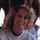# Algebra question: How does 2^-2=1/4? Why doesn't it equal -4?

Asked by Dutchess_III (43263) July 7th, 2010

Broken down the equation reads 2 * -2, right? Which which equals -4, right? So how do they get ¼?

Observing members: 0Composing members: 0## 16 Answers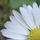Allie (17506)“Great Answer” (0) Flag as…….why?

Dutchess_III (43263)“Great Answer” (0) Flag as…(Click the link.)

Allie (17506)“Great Answer” (0) Flag as…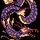You’re confusing the carrot with the multiplication sign.

2 TIMES -2 is equal to -4.

2 raised to the power of -2 is an entirely different matter.

Qingu (21175)“Great Answer” (0) Flag as…@Qingu I did not confuse the carrot with the multiplication sign.

@Allie .... It’s starting to click….. let me look some more. Thank you…..That purple math is a really nice, user-friendly website, isn’t it!

Dutchess_III (43263)“Great Answer” (0) Flag as…@Dutchess_III, you said, “broken down the equation reads 2 * -2, right?”

How did you break it down?

Qingu (21175)“Great Answer” (0) Flag as…Dang it. I’ve been teaching an “on line” class for a few months now. I’m going to be taking an online class this fall to refresh my algebra skills…but it just isn’t the same as having a live person to talk to…..

@Qingu because, for example, 2^6= 2 X 2 X2 X 2 X 2 X 2. That’s how I was trying to look at it, but I have to figure out why I need to look at it differently since the exponent is a negative..

Dutchess_III (43263)“Great Answer” (0) Flag as…Here’s another way to think of it.

What happens when you subtract 1 from an exponent?

Like, what is the difference between x^3 and x^2?

x^3, divided by x, equals x^2.

x^2, divided by x, equals x^1 (which is just plain ol’ x).

x^1, divided by x, equals x^0 (which is just x/x, or 1.)

x^0, divided by x, equals x^-1… or 1/x.

And so on.

The exponent becomes negative… but all you’re doing is dividing by x more often. You’re not multiplying by a negative.

Qingu (21175)“Great Answer” (0) Flag as…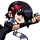2^(-2) = 1/(2^2)

It’s just notation.

Ivan (13479)“Great Answer” (1) Flag as…Hey @Ivan! So 2^2 would be 2^2/1 (4/1) but the negative exponent flips the equation to ½^2 (¼)...right? That’s simplistic, but I can remember it….I just have to remember the “rules,” right? Rules that were figured out by people way more ‘marter than me…

@Qingu Thank you….that’s the way my mind was starting to meander. And I have a headache.

Dutchess_III (43263)“Great Answer” (0) Flag as…@Dutchess_III

I guess you can think of it that way, but I think you’ll find it less confusing in the long run to think of it in the way that I expressed it in my earlier comment.

Ivan (13479)“Great Answer” (0) Flag as…Like, “It’s just a notation. Don’t mean nuthin’”?

Dutchess_III (43263)“Great Answer” (0) Flag as…No, I mean, when you see a negative exponent in the numerator, just stick it in the denominator with a positive exponent.

Ivan (13479)“Great Answer” (1) Flag as…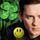Think of it as x (times) if it is positive and / (divide) if it is negative
2^4 = 1×2x2×2x2 = 16
2^-4= 1 /2/2/2/2= 1/16

LuckyGuy (39116)“Great Answer” (0) Flag as…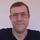Maybe it helps to know that x^0 is always 1, even 0^0. So 2^0=1

2^∞=∞
...
2^2=4
2^1=2
2^0=1
2^-1=½
2^-2=¼
...
2^-∞=0

mattbrowne (31643)“Great Answer” (0) Flag as…Thanks very much guys!

Dutchess_III (43263)“Great Answer” (0) Flag as…## Answer this questionor

#### Join

to answer.
Your answer will be saved while you login or join.
Have a question? Ask Fluther!
What do you know more about?
or
Knowledge Networking @ Fluther